# GeoMaestro

-- Back --

## Distortion Functions Reference

Here are illustrated some of the provided distortion functions. If you studied maths, you will find them very basic. If you did not, it should give you an idea of what they do.

For each of the folloing pictures, the source event is located at 0,0 and its nodur is ´a´, that is a MIDI 69 note with volume 63.

The 3-D plots where computed by Scilab

`PitPlouf`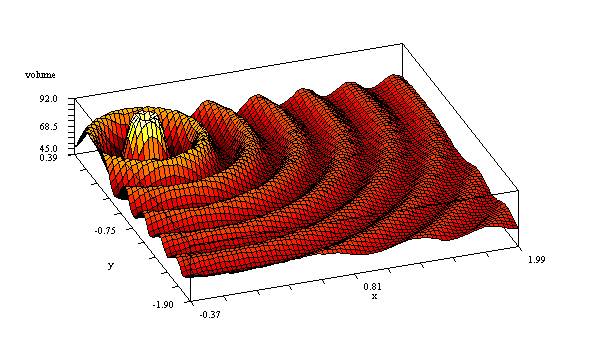p1 = 0.05, p2 = 80

`VolIsAngle`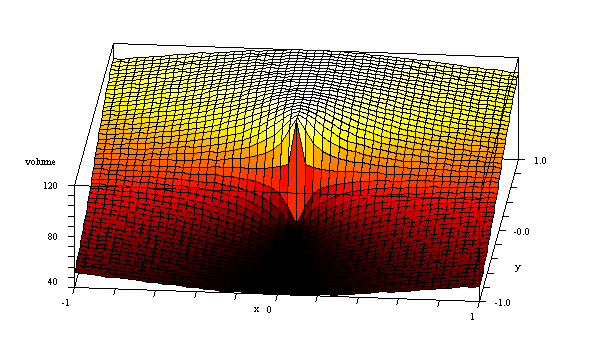p1=40, p2=120, p3=0

`Vexp`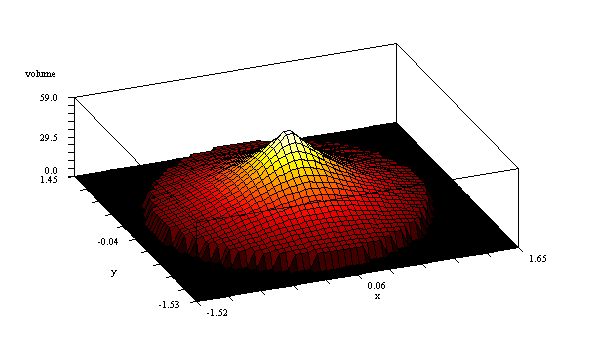p1=0.5

`OctaveRings`p1=0.05, p2=100

`PlusSineN`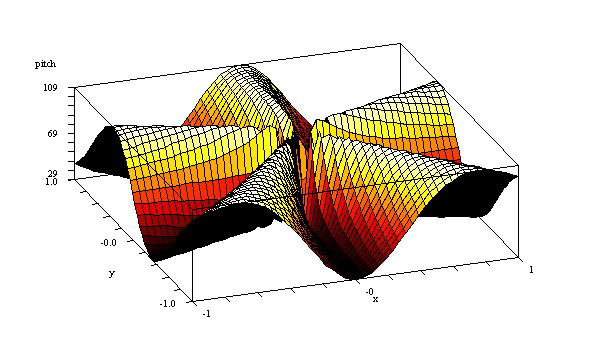p1=40, p2=5, p3=0

`PlusTriN`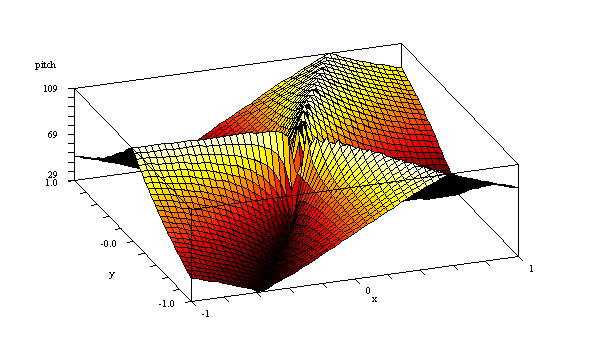p1=40, p2=3

`AigusAuLoin`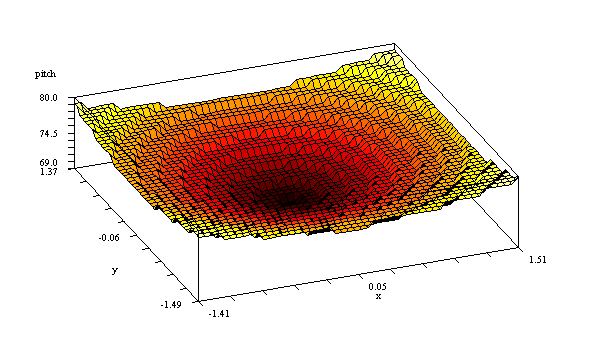p1=0.2, p2=120

`BassesAuLoin`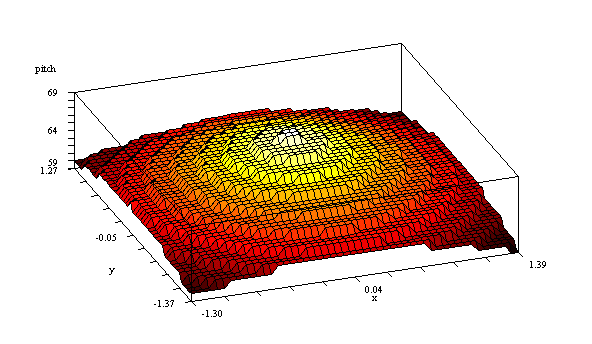p1=0.2, p2=120

-- Back --